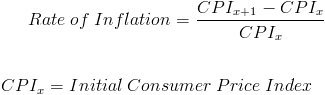# Rate of InflationThe rate of inflation formula measures the percentage change in purchasing power of a particular currency. As the cost of prices increase, the purchasing power of the currency decreases.

The rate of inflation formula shown uses the Consumer Price Index which is released by the Bureau of Labor Statistics in the US. However, other similar indices may be used at times. If another index is used, "CPI" in the rate of inflation formula is replaced by the alternative index.

The subscript "x" refers to the initial consumer price index for the period being calculated, or time x. And such, subscript "x+1" would be the ending consumer price index for the period calculated, or time x+1.

## Use of Rate of Inflation Formula

The formula for the rate of inflation is primarily used by economists. On the financial side, the rate of inflation may be used by corporations to compare expenses, revenues, and profit across multiple years.

The rate of inflation formula shown is not to be confused with the purchasing power of goods relative to income.
An example, albeit an extreme example, would be an individual who recently discovers that their income will increase to \$1,000,000 from \$20,000 per year--a 5,000% increase. The individual, unable to hold back excitement, decides to go shopping only to discover that a loaf of bread suddenly increased to \$300 from \$3--a 10,000% increase. The same result occurs as the individual purchases more products. Soon the individual realizes that they are worse off than prior to the income change. The rate of inflation formula measures only inflation, the 10,000% price increase in the example, and does not consider income, the 5,000% income increase in the example, or standard of living.

## Annualizing the Rate of Inflation Formula

As with annualizing any monthly rate, the monthly rate of inflation can not be annualized by simply multiplying it by 12, as this does not consider compounding. The same concept can be applied to adding each monthly percentage change in the consumer price index as an attempt to find the annual percentage change in the consumer price index. The proper way to calculate the annual rate of inflation is to use the year's initial and ending CPI in the formula.

New to Finance?